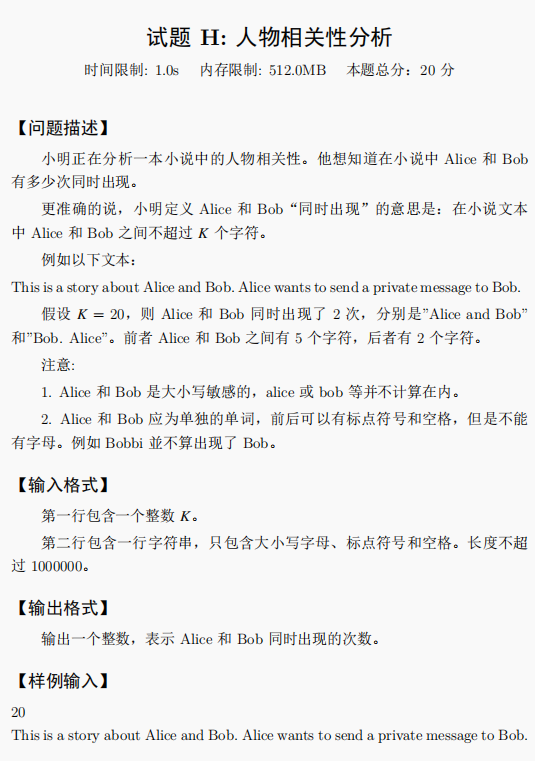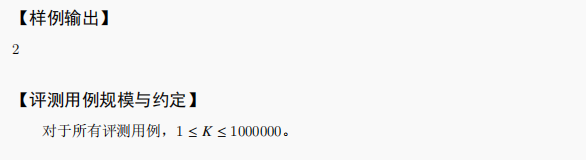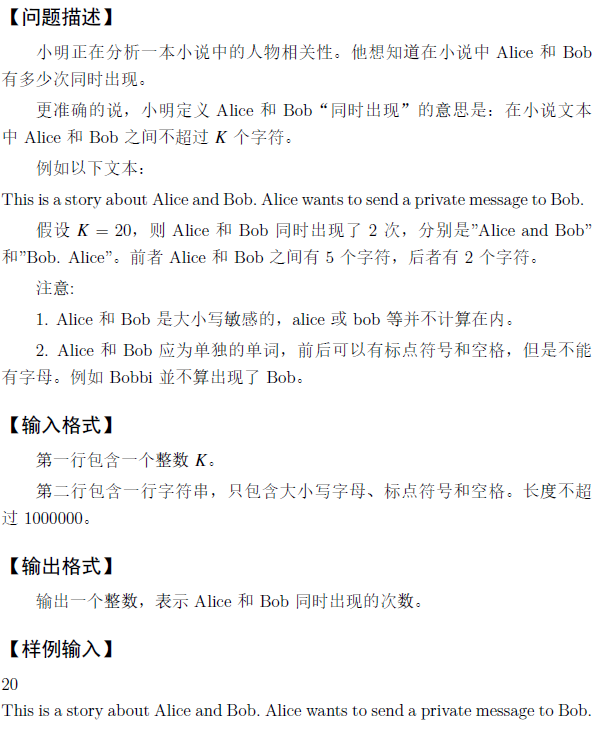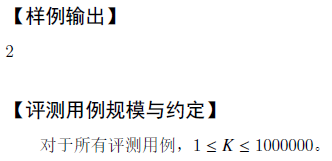• Java实现第十届蓝桥杯人物相关性分析
万次阅读 多人点赞
2019-07-27 22:18:51

试题 H: 人物相关性分析
时间限制: 1.0s 内存限制: 512.0MB 本题总分：20 分
【问题描述】
小明正在分析一本小说中的人物相关性。他想知道在小说中 Alice 和 Bob 有多少次同时出现。 更准确的说，小明定义 Alice 和 Bob“同时出现”的意思是：在小说文本 中 Alice 和 Bob 之间不超过 K 个字符。 例如以下文本： ThisisastoryaboutAliceandBob.AlicewantstosendaprivatemessagetoBob. 假设 K = 20，则 Alice 和 Bob 同时出现了 2 次，分别是”Alice and Bob” 和”Bob. Alice”。前者 Alice 和 Bob 之间有 5 个字符，后者有 2 个字符。 注意: 1. Alice 和 Bob 是大小写敏感的，alice 或 bob 等并不计算在内。 2. Alice 和 Bob 应为单独的单词，前后可以有标点符号和空格，但是不能 有字母。例如 Bobbi 並不算出现了 Bob。
【输入格式】
第一行包含一个整数 K。 第二行包含一行字符串，只包含大小写字母、标点符号和空格。长度不超 过 1000000。
【输出格式】
输出一个整数，表示 Alice 和 Bob 同时出现的次数。
【样例输入】
20 This is a story about Alice and Bob.Alice wants to send aprivate message to Bob.

这道题最大的坑在于，你直接从PDF中复制下来， 他会没有空格，很多人做的时候会忽视这一点
import java.util.Scanner;

public class renwuxiangguanxing {
public static void main(String[] args)  {
int res=0;    //save result
String words[]=str.split("\\s+|\\.");    //以空格和.分割出来，注意.空格的组合存放为空字符串

//    Alice------>Bob
for(int i=0;i<words.length;i++){
if(words[i].equals("Alice")){
for(int j=i+1;j<words.length;j++){
if(words[j].equals("Bob")){
int sum=1;    //这里要等于1
for(int k=i+1;k<j;k++){
sum+=words[k].length()+1;
}
if(sum<=K){
res++;
}
}
}
}
}

//Bob--------->Alice
for(int i=0;i<words.length;i++){
if(words[i].equals("Bob")){
for(int j=i+1;j<words.length;j++){
if(words[j].equals("Alice")){
int sum=1;    //这里要等于1
for(int k=i+1;k<j;k++){
sum+=words[k].length()+1;
}
if(sum<=K){
res++;
}
}
}
}
}
System.out.println(res);
}

}Java
更多相关内容
• 这里写自定义目录标题题目：分析：AC代码： 题目： 20 This is a story about Alice and Bob. Alice wants to send a private message to Bob 分析： 字符串长度一百万，肯定不能挨个枚举。 所以，我们可以枚举...

# 题目：20
This is a story about Alice and Bob. Alice wants to send a private message to Bob# 分析：

字符串长度一百万，肯定不能挨个枚举。
所以，我们可以枚举区间，
首先，我们找出所有的Alice放在Alice集合内
然后，我们找出所有的Bob放在Bob集合内
最后，我们只需要找出每个人名字前面k的区间内有几个另一个人的名字。
区间内人名数量的和就是答案！

为什么只算前面不算后边？

距离是相互的，A到B的距离等于B到A的距离，如果前后计算就重复了。

# AC代码：

package JC2019;

import java.util.Scanner;
import java.util.Vector;

public class H {
static boolean check(char C){
if ((C>='a'&&C<='z')||(C>='A'&&C<='Z')) {
return false;
}
return true;
}
public static void main(String[] args) {
Vector<Integer> Alice = new Vector<Integer>();
Vector<Integer> Bob = new Vector<Integer>();

Scanner sc = new Scanner(System.in);
int n = sc.nextInt();
String s = sc.nextLine();
s = sc.nextLine();

for (int i = 0; i+5 <= s.length(); i++) {
if ((i==0 || check(s.charAt(i-1))) && (i+5==s.length() || check(s.charAt(i+5)))) {
if (s.substring(i, i+5).equals("Alice")) {
}
}
}
for (int i = 0; i+3 <= s.length(); i++) {
if ((i==0 || check(s.charAt(i-1))) && (i+3==s.length() || check(s.charAt(i+3)))) {
if (s.substring(i, i+3).equals("Bob")) {
}
}
}
int ans = 0;
for (int i = 0; i < Alice.size(); i++) {
int l =0,r=-1;
while(r+1 < Bob.size() && Alice.get(i) > Bob.get(r+1)  ) {
r++;
}
while (l<=r && Alice.get(i) >Bob.get(l)+n+3  ) {
l++;
}
ans += r-l+1;
}

for (int i = 0; i < Bob.size(); i++) {
int l  =0,r=-1;
while(r+1 < Alice.size() && Bob.get(i) > Alice.get(r+1)  ) {
r++;
}
while (l<=r && Bob.get(i) >Alice.get(l)+n+5  ) {
l++;
}
ans += r-l+1;
}
System.out.println(ans);
}
}


展开全文Java 算法
• 资源限制 时间限制：1.0s 内存限制：256.0MB 思路： 按照平常思考，我们会用枚举，先计算字符串中出现的每一个Alice的位置，利用双重循环计算每个Alice与每一个Bob的位置，当两位置相差不到K，则计数加一。...

资源限制

时间限制：1.0s   内存限制：256.0MB## 思路：

按照平常思考，我们会用枚举，先计算字符串中出现的每一个Alice的位置，利用双重循环计算每个Alice与每一个Bob的位置，当两位置相差不到K，则计数加一。但这样的方法时间复杂度可达到10^12 ，必定超时。

所以需要灵活运用枚举，稍稍改变一下思路。

我们可以考虑当发现第一个Alice时，位置记作A1，则当Bob出现在[A1-K-3,A1+5+K]范围内时，

(-3是因为Bob长度为3，Alice长度为5，每次只记录A和B的下标)Bob出现几个就记多少次。（这个范围是因为题中按字符来算，我们需要考虑Alice和Bob的字符长度）

同理，出现第二个Alice时，位置记作A2，按照上面相同的方法计算出现多少次。

这样就从遍历全部字符串改变成遍历一个移动区间。
参考：https://blog.csdn.net/weixin_46259848/article/details/123167845

## Code

#include<bits/stdc++.h>
char s;
int numa = 0, numb = 0;//a,b的个数
int i, j, k;
long alice;//alice[i]存的是alice第i+1出现的位置
long bob;//同理
int ans, rp, lp;
int main() {

scanf("%d\n", &k);//'\n'吸收换行符，防止换行符留在缓冲区中被s录入
scanf("%s", s);
for (i = 0; i < strlen(s); i++) {
if ((i - 1 < 0 || s[i - 1] == '.' || s[i - 1] == ' ') && s[i] == 'A' && s[i + 1] == 'l' && s[i + 2] == 'i' && s[i + 3] == 'c' && s[i + 4] == 'e' && (s[i + 5] == '.' || s[i + 5] == ' ')) {
alice[numa] = i;//Alice的前面如果是非法/./空格，说明它是头一次被计数
numa++;
}
if ((i - 1 < 0 || s[i - 1] == '.' || s[i - 1] == ' ') && s[i] == 'B' && s[i + 1] == 'o' && s[i + 2] == 'b' && (s[i + 3] == '.' || s[i + 3] == ' ')) {
bob[numb] = i;//同理
numb++;
}
}
for (j = 0; j < numa; j++) {
while (bob[lp] < alice[j] - k - 3)lp++;//如果左边Bob的位置不在区间内，则将下一个Bob的位置与区间进行比对，直到区间中有第一个Bob
while (bob[rp + 1] <= alice[j] + k + 5)rp++;//如果右边Bob的位置还在区间内，可尝试看下一个Bob是否也在区间内，直到下一个Bob的位置不在区间内，则区间中的Bob数就可以用rp-lp+1得出
if (rp - lp + 1 > 0)
ans += (rp - lp + 1);
}
printf("%d", ans);
return 0;
}


展开全文算法
• 用string.find()直接枚举，能拿45分不知道是哪里错误了，如果请大佬指出，如果对string.find的时间复杂度有质疑，请问度娘 #include <bits/stdc++.h> using namespace std; int cnt,k; bool temp = true;...

用string.find()直接枚举，能拿45分不知道是哪里错误了，如果请大佬指出，如果对string.find的时间复杂度有质疑，请问度娘

#include <bits/stdc++.h>
using namespace std;
int cnt,k;
bool temp = true;
char f = {"Alice", "Bob"};
bool check(string &a, int &pa, int &pb)//pa指的是之前Alice出现的位置
{
bool flage = true;
pa = a.find(f, ++pa);
if (pa == -1)
temp = false;
if(temp&&abs((pa-1) - pb) < k&&pb)//这里加一条这个就是因为相对位置的关系
cnt++;
if (a[pa - 1] != ' ' && a[pa + 6] != ' ')
flage = false;
pb = a.find(f, ++pb);//++pb是因为不加的话会一直查找同一子串
if (pb == -1)
temp = false;
if (a[pb - 1] != ' ' && a[pb + 6] != ' ')
flage = false;
return flage;
}
int main()
{
string a;
cin >> k;
getchar();//吃个回车
getline(cin, a);//用cin会卡空格，getline就完全没有这些担忧
int pa = 0, pb = 0;
while (1)
{
if (check(a, pa, pb) && abs(pa - pb) < k && temp)
{
cnt++;
}
else if (!temp)//如果没查到任意一个我们就直接退出
break;
}
cout << cnt;
return 0;
}


下面我决定换个思路用滑动窗口做这题
这边介绍一下滑动窗口，主要就是用来解决一些满足一定条件的连续区间性质的问题有兴趣的可以自己问问度娘

#include <bits/stdc++.h>
using namespace std;
int len;
string s;
bool check(int i)
{
if (len - i < 5) return false;
return s[i + 1] == 'l' && s[i + 2] == 'i' && s[i + 3] == 'c' && s[i + 4] == 'e';
}
bool check2(int i)
{
if (len - i < 3)
return false;
return s[i + 1] == 'o' && s[i + 2] == 'b';
}
int main()
{
int k;//这里绝对不能加关闭流读入，如果这加了getline会直接读不到
cin >> k;
getchar();
getline(cin, s);
len = s.length();
vector<int> Alice, Bob;
for (int i = 0; i < len; i++)
{
if (s[i] == 'A' && check(i))
{
Alice.push_back(i);
i += 5;
}
else if (s[i] == 'B' && check2(i))
{
Bob.push_back(i);
i += 3;
}
}
int As = Alice.size(), Bs = Bob.size();
int i = 0, j = 0;
long long ans = 0;
for (int q = 0; q < As; q++)
{
while (i < Bs && Bob[i] < Alice[q] - k - 3)
i++; //左边界已经有些被排除的
while (j < Bs && Bob[j] <= Alice[q] + k + 5)
j++; //右边界
ans += j - i;
}
cout << ans << "\n";
return 0;
}

展开全文• 小明正在分析一本小说中的人物相关性。 他想知道在小说中 Alice 和 Bob 有多少次同时出现。 更准确的说，小明定义 Alice 和 Bob “同时出现”的意思是：在小说文本中 Alice 和 Bob 之间不超过 K 个字符。 例如以下...
• 人物相关性分析 【问题描述】 小明正在分析一本小说中的人物相关性。他想知道在小说中 Alice 和 Bob 有多少次同时出现。 更准确的说，小明定义 Alice 和 Bob“同时出现”的意思是：在小说文本 中 Alice 和 Bob ...算法
• 思路： 这道题是常规的模拟题，根据题意写出相关代码即可。模拟题一般容易在边界条件上出错，建议自己设计几个样例测试一下。这题纯暴力的方法不能通过所有的测试点，对于最后的查询，应该使用二分查找，这样算法的...
• 蓝桥杯人物相关性分析 题解： 最容易想到的便是枚举遍历，但由于数量巨大必定会超时。 因此我们可考虑使用双指针来模拟滑动窗口解决该题。 首先我们创建两个数组用双指针查找分别存储出现的Alice和Bob的头部下标...指针 字符串 数据结构 哈希
• 小明 正在分析一本小说中的人物相关性。他想知道小说中Alice和Bob有多少次同时出现 更准确的说，小明定义Alice和Bob同时出现的意思是，在小说文本你中Alice和Bob之间不超过K个字符 注意 Alice和Bob是大小写敏感的，...字符串 正则表达式 leetcode
• 题目描述题目描述小明正在分析一本小说中的人物相关性。他想知道在小说中 Alice 和 Bob 有多少次同时出现。更准确的说，小明定义 Alice 和 Bob“同时出现”的意思是：在小说文本 中 Alice 和 Bob 之间不超过 K 个...
• importjava.util.List;importjava.util.ArrayList;importjava.util.Scanner;importjavax.swing.text.DefaultEditorKit.InsertBreakAction;public classMain{static List list = new ArrayList();...
• Java试题 H: 人物相关性分析 【问题描述】 小明正在分析一本小说中的人物相关性。他想知道在小说中 Alice 和 Bob 有多少次同时出现。 更准确的说，小明定义 Alice 和 Bob“同时出现”的意思是：在小说文本 中 Alice ...java
• 人物相关性分析 2019年 【问题描述】 小明正在分析一本小说中的人物相关性。 他想知道在小说中 Alice 和 Bob 有多少次同时出现。 更准确的说， 小明定义 Alice 和 Bob “同时出现”的意思是：在小说文本中 Alice 和 ...c语言
• 试题 H: 人物相关性分析 时间限制: 1.0s 内存限制: 512.0MB 本题总分：20 分 【问题描述】 小明正在分析一本小说中的人物相关性。他想知道在小说中 Alice 和 Bob 有多少次同时出现。 更准确的说，小明定义 Alice 和 ...字符串 java
• 题目： 第八题：人物相关性分析 题目描述 小明正在分析一本小说中的人物相关性。他想知道在小说中 Alice 和 Bob 有多少次同时出现。 更准确的说，小明定义 Alice 和 Bob“同时出现”的意思是：在小说文本 中 Alice ...java
• 题目： 这个题在蓝桥杯官网案例错误，所以没法判断代码得分。 思路：用两个list数组分别记录这两个字符串出现的位置，...public class H_人物相关性分析 { public static void main(String[] args) { Scanner sc =java leetcode 动态规划
• 小明正在分析一本小说中的人物相关性。他想知道在小说中 Alice 和 Bob 有多少次同时出现。 更准确的说，小明定义 Alice 和 Bob“同时出现”的意思是:在小说文本 中 Alice 和 Bob 之间不超过 K 个字符。 例如以下文本...数据结构 算法
• 试题 H: 人物相关性分析 时间限制: 1.0s 内存限制: 512.0MB 本题总分：20 分 【问题描述】 小明正在分析一本小说中的人物相关性。他想知道在小说中 Alice 和 Bob 有多少次同时出现。 更准确的说，小明定义 Alice 和 ...
• 小明正在分析一本小说中的人物相关性。他想知道在小说中 Alice 和 Bob 有多少次同时出现。 更准确的说，小明定义 Alice 和 Bob“同时出现”的意思是： 在小说文本 中 Alice 和 Bob 之间不超过 K 个字符。 例如以下...
• 1473: [蓝桥杯2019初赛]人物相关性分析 题目描述 输入 输出 样例输入 20 This is a story about Alice and Bob. Alice wants to send a private message to Bob. 样例输出 2 解题思路 根据字符串特性，...算法
• 小明正在分析一本小说中的人物相关性。他想知道在小说中 Alice 和 Bob 有多少次同时出现。 更准确的说，小明定义 Aice 和Bob“同时出现” 的意思是：在小说文本 中 Alice 和Bob之间不超过下个字符。 例如以下文本： ...java 算法
• /** * 试题H：人物相关性分析 8 Bob Alice sdf Bob Alice hgdgBob Alice gasdBob Alice Bob Alice asd BobAlice * */ public class Correlation { static Scanner sc = new Scanner(System.in); static int K =...Java算法
• 小明正在分析一本小说中的人物相关性。他想知道在小说中 Alice 和 Bob 有多少次同时出现。 更准确的说，小明定义 Alice 和 Bob“同时出现”的意思是：在小说文本 中 Alice 和 Bob 之间不超过 K 个字符。 例如以下...java 数据结构 算法 leetcode
• 【题目】人物相关性分析 思路：我是这么觉得：先把字符串按空格分割成String[]，把"Alice"和"Bob"和"Alice."和"Bob."放到HashMap的键上。每次去比较就行，我感觉这样就简单点了。我写了，但是蓝桥杯的样例太古怪了...java...